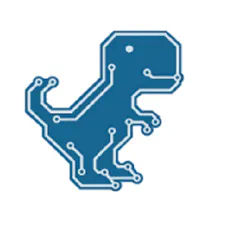Speed Measurement Using HC-SR04 Ultrasonic Sensor

On this project I will show you how to measure the speed of movement of an object using HC-SR04 ultrasonic sensor.

BeginnerFull instructions provided9,424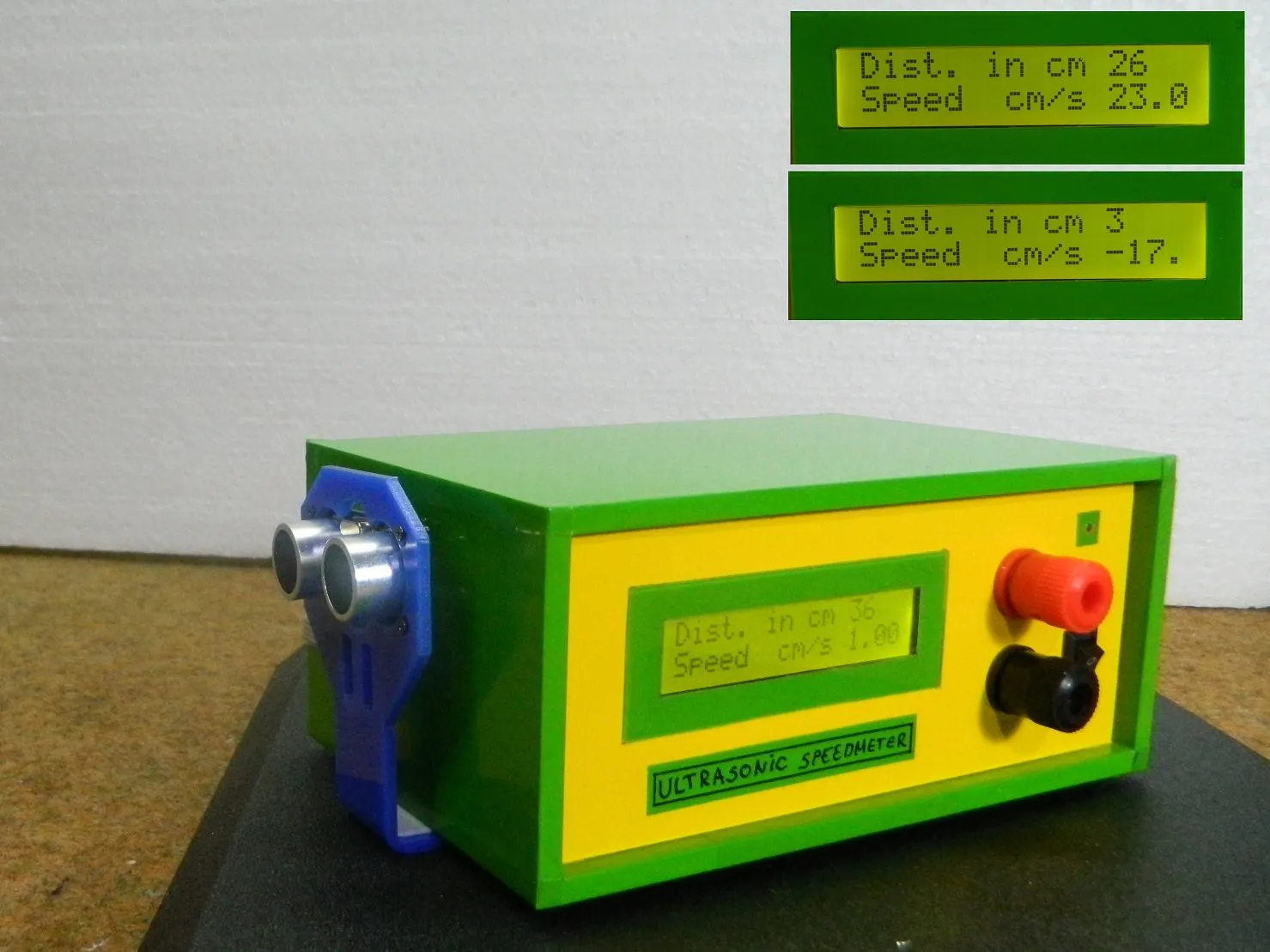Things used in this project

Hardware componentsArduino Nano R3
×1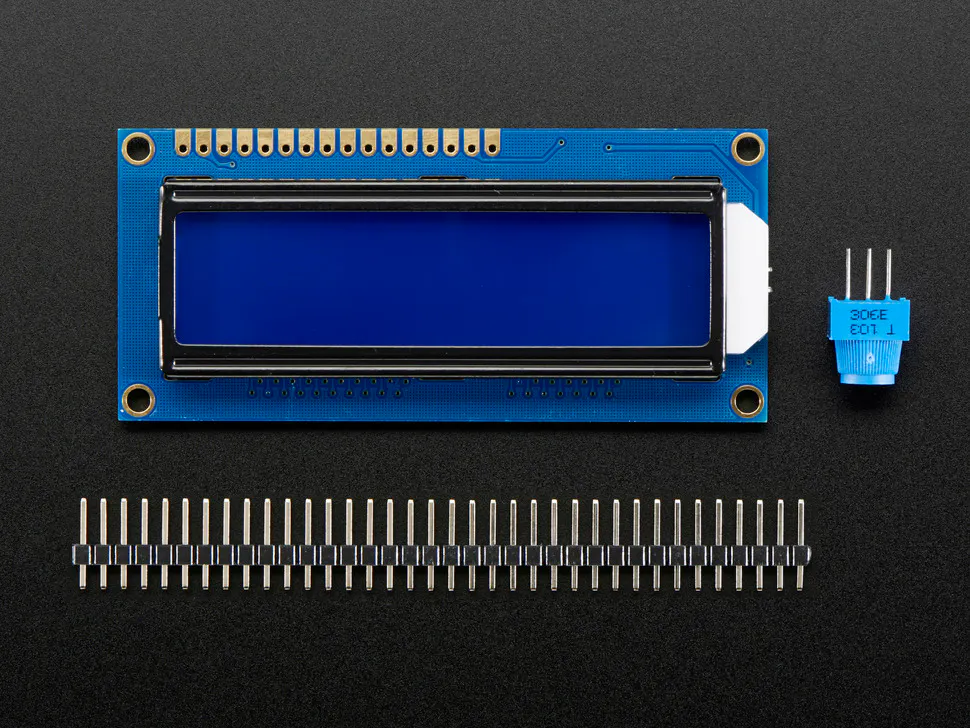Adafruit Standard LCD - 16x2 White on Blue
×1Ultrasonic Sensor - HC-SR04 (Generic)
×1LED (generic)
×1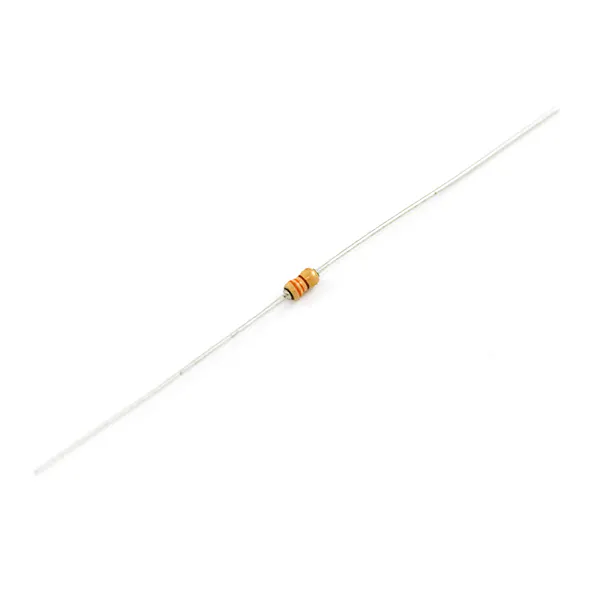Resistor 330 ohm
×1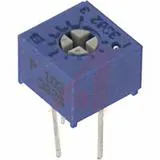Single Turn Potentiometer- 10k ohms
×1

Software apps and online servicesArduino IDE

Hand tools and fabrication machinesSoldering iron (generic)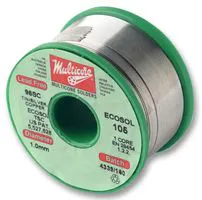Schematics

Schematic diagram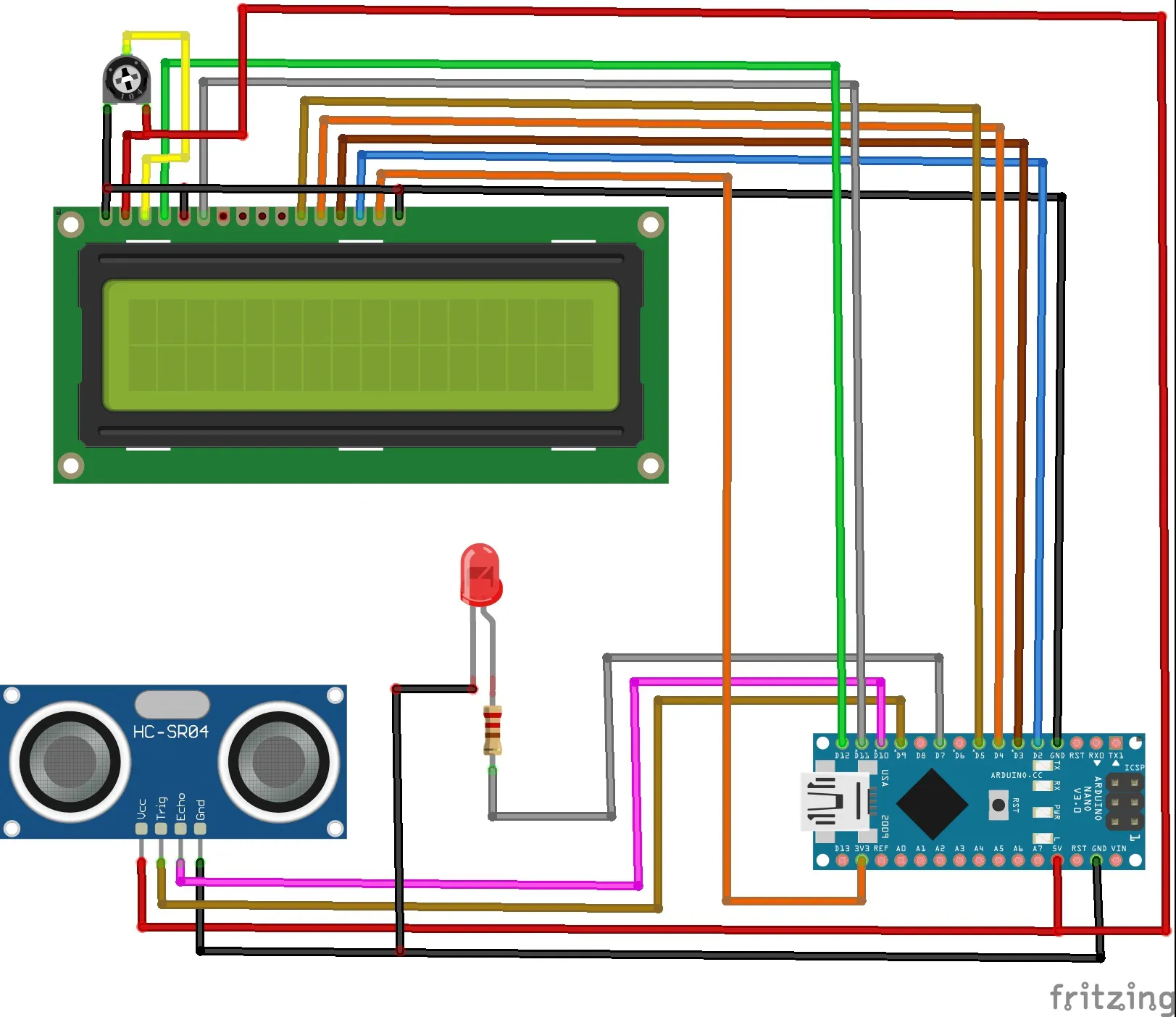Code

Arduino code

C/C++
#include <LiquidCrystal.h>
LiquidCrystal lcd(12, 11, 5, 4, 3, 2);// RS,E,D4,D5,D6,D7
// defines pins numbers
const int trigPin = 9;
const int echoPin = 10;

// defines variables
long duration;
int distance1=0;
int distance2=0;
double Speed=0;
int distance=0;

void setup()
{
lcd.begin(16, 2);// LCD 16X2
pinMode(trigPin, OUTPUT); // Sets the trigPin as an Output
pinMode(echoPin, INPUT); // Sets the echoPin as an Input
pinMode( 7 , OUTPUT);
Serial.begin(9600); // Starts the serial communication
}

void loop()
{

//calculating Speed

delay(1000);//giving a time gap of 1 sec

//formula change in distance divided by change in time
Speed = (distance2 - distance1)/1.0; //as the time gap is 1 sec we divide it by 1.

//Displaying Speed
Serial.print("Speed in cm/s  :");
Serial.println(Speed);
lcd.setCursor(0,1);
lcd.print("Speed  cm/s ");
lcd.print(Speed);

// LED indicator
if (distance >0 && distance <5)
{
digitalWrite( 7 , HIGH);
delay(50);                  // waits for a second
}

if (distance > 5 && distance <10 )
{
digitalWrite( 7 , HIGH);
delay(50);                  // waits for a second
digitalWrite( 7 , LOW);    // sets the LED off
delay(50);                  // waits for a second
}

if (distance >10  && distance < 20)
{
digitalWrite( 7 , HIGH);
delay(210);                  // waits for a second
digitalWrite( 7 , LOW);    // sets the LED off
delay(210);                  // waits for a second
}

if (distance >20  && distance < 35)
{
digitalWrite( 7 , HIGH);
delay(610);                  // waits for a second
digitalWrite( 7 , LOW);    // sets the LED off
delay(610);                  // waits for a second
}

}

{
// Clears the trigPin
digitalWrite(trigPin, LOW);
delayMicroseconds(2);

// Sets the trigPin on HIGH state for 10 micro seconds
digitalWrite(trigPin, HIGH);
delayMicroseconds(10);
digitalWrite(trigPin, LOW);

// Reads the echoPin, returns the sound wave travel time in microseconds
duration = pulseIn(echoPin, HIGH);

//calculating distance
distance= duration*0.034/2;

// Prints the distance on the Serial Monitor
Serial.print("Distance in cm : ");
Serial.println(distance);
lcd.setCursor(0,0);
lcd.print("Dist. in cm ");
lcd.print(distance);
lcd.print("   ");
return distance;

}